# Properties of Circle

Lines and circles are the important elementary figures in geometry. We know that a line is a locus of a point moving in a constant direction, whereas the circle is a locus of a point moving at a constant distance from some fixed point. The theoretical importance of the circle is reflected in the number of amazing applications. Here we will discuss the properties of a circle, area and circumference of a circle in detail.

## Circle Definition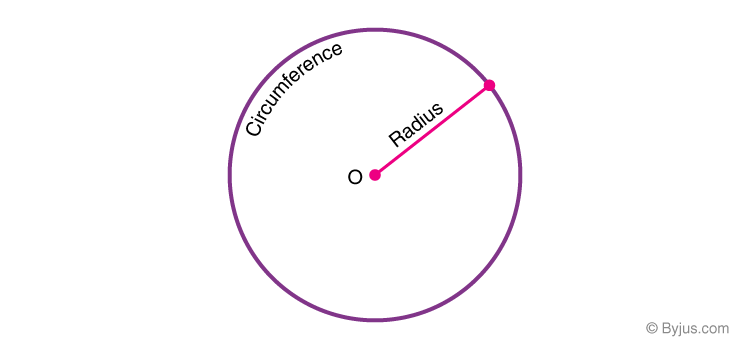The collection of all the points in a plane, which are at a fixed distance from a fixed point in the plane, is called a circle. Here, the fixed point is called the centre “O”. Some of the important terminologies used in the circle are as follows: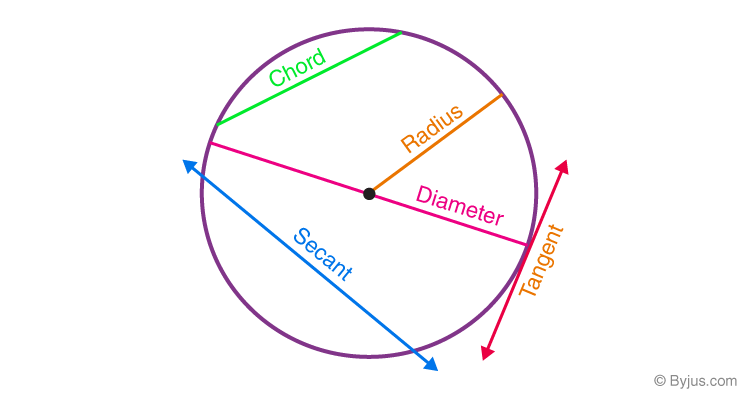Terms Description Circumference The boundary of the circle is known as the circumference Radius The line from the centre “O” of the circle to the circumference of the circle is called the radius and it is denoted by “R” or “r” Diameter The line that passes through the centre of the circle and touches the two points on the circumference is called the diameter and it is denoted by the symbol “D” or “d” Arc Arc is the part of the circumference where the largest arc is called the major arc and the smaller one is called the minor arc Sector Sector is slice of a circle bounded by two radii and the included arc of a circle Chord The straight line that joins any two points on the circumference of a circle is called the chord Tangent A line that touches the circumference of a circle at a point is called the tangent Secant A line that cuts the circle at the two distinct points is known as the secant

## Circle Properties

Some of the important properties of the circle are as follows:

• The circles are said to be congruent if they have equal radii
• The diameter of a circle is the longest chord of a circle
• Equal chords of a circle subtend equal angles at the centre
• The radius drawn perpendicular to the chord bisects the chord
• Circles having different radius are similar
• A circle can circumscribe a rectangle, trapezium, triangle, square, kite
• A circle can be inscribed inside a square, triangle and kite
• The chords that are equidistant from the centre are equal in length
• The distance from the centre of the circle to the longest chord (diameter) is zero
• The perpendicular distance from the centre of the circle decreases when the length of the chord increases
• If the tangents are drawn at the end of the diameter, they are parallel to each other
• An isosceles triangle is formed when the radii joining the ends of a chord to the centre of a circle

## Circle Formulas

Area of a circle, A = πr2 square units

The circumference of a circle = 2πr units

The circumference of a circle formula can also be written as πd.

Where,

d = 2r

Here “r” represents the radius of a circle.

## Video Lessons on Circles

### Introduction to Circles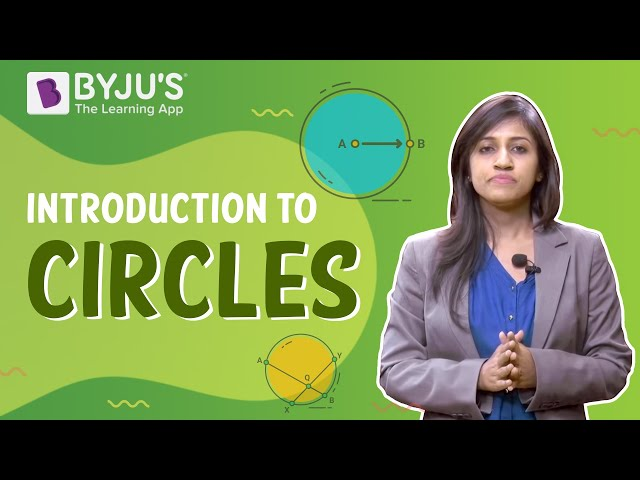### Parts of a Circle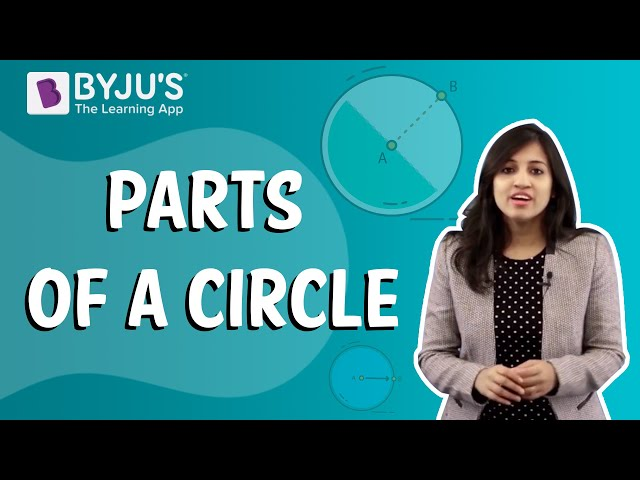### Area of a Circle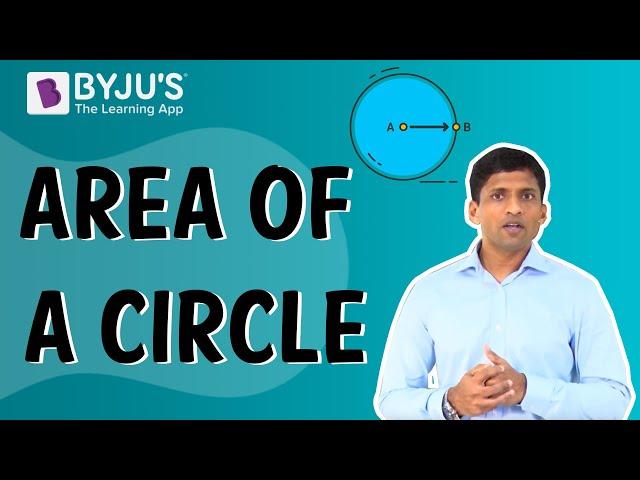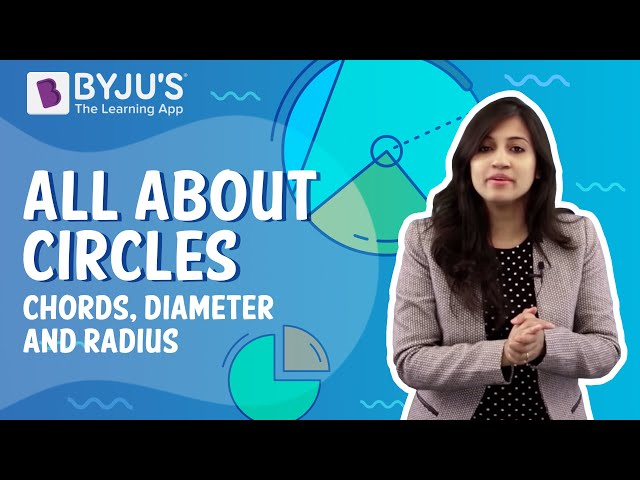## Circle Solved Problems

The sample examples to find the area and circumference of a circle is given below.

Example 1:

Find the area and circumference of a circle having the diameter value of 10 cm.

Solution:

Given:

Diameter, d = 10 cm

We know that diameter = 2 x Radius

r = 10/2 = 5

So, the radius is 5 cm.

Area of a circle, A = πr2 square units

A = 3.14 x 5 x 5

Where ,

π = 3.14

A = 3.14 x 25

A = 78.5 cm2

Therefore, the area of a circle is 78.5 square units

The circumference of a circle = 2πr units

C = 2 x 3.14 x 5

C = 10 x 3.14

C = 31. 4 cm

Therefore, the circumference of a circle is 31.4 units.

Example 2:

A circle is cut out from a square paper of side 7 cm, if the diameter of the circle is equal to the side of the square, find how much paper is left after cutting out the circle.

Solution:

Side of square = 7 cm

Diameter of circle = 7 cm

Radius of circle = 7/2 cm

Paper left after cutting out the circle = Area of square paper – Area of circle

= side2– (𝜋r2) = 72– [22/7 × (7/2)2]

= 49 – 38.5

= 10.5 cm2

Example 3:

Find the distance covered by a wheel of radius 56 cm in 20 revolutions.

Solution:

Radius of wheel = 56 cm

Circumference of wheel = 2𝜋r = 2 × 22/7 × 56 = 352 cm

Distance covered in 20 revolutions = 20 × 352 = 7040 cm = 70.40 m

## Practice Questions

1. Find the radius of the circle whose area is 1386 cm2.
2. Find the area swept by the minute hand of length 7 cm of the clock in an hour.
3. Find the area of a circle if the circumference of the circle is  31.4 m2.

For more interesting information on the properties of a circle, register with BYJU’S – The Learning App and also watch videos to learn with ease.

## Frequently Asked Questions on Properties of Circle

### What is the condition for the congruency of circles?

If the given circles have equal radii then the circles are congruent.

### What is the condition for the similarity of circles?

Every circle is similar to each other irrespective of its radii.

### What are the properties of the tangent to the circle?

Following are the properties of the tangent to the circle:
1) Radius of the circle is perpendicular to point of contact between the tangent and the circle.
2) Tangents drawn from an external point of the circle are of equal length.
3) Tangents drawn from an external point are equally inclined to the line segment joining the external point and centre of the circle.
4) Tangents drawn at the endpoints of the diameter of a circle are parallel to each other.

### What is a characteristic of a circle?

A circle is a geometrical figure formed by the locus of points which are equidistant to a common point called the centre of the circle, and the constant distance from the centre is called the radius of the circle.

Quiz on Properties of Circle